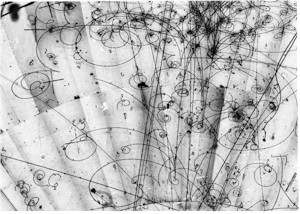# Physics Hint 1The bubble chamber is a charged particle detector used in conjunction with a high-energy particle accelerator. Particle interactions initiated by the accelerator are observed in the bubble chamber, which consists of a superheated liquid, i.e. a liquid heated to a temperature slightly above its boiling point, in this case liquid hydrogen. Such a state is metastable - a small disturbance in the liquid will initiate boiling. A charged elementary particle such as an electron or proton is sufficient to initiate the boiling process, so leaves a stream of tiny bubbles in its wake, which can then be photographed from several angles. The three dimensional tracks are then determined by geometrical methods. The bubble chamber is surrounded by a large magnet, which forces the charged particles to follow curved spiral trajectories. This aids in particle identification, as positive and negative particles will curve oppositely in the magnetic field, and the radius of curvature is proportional to the particle's momentum (technically the momentum component perpendicular to the field). Particles lose energy slowly through collisions as they traverse the liquid, so they spiral inward with progressively tighter radii of curvature. The rate of energy loss also helps to indentify the types of particles involved.

Assuming the magnetic field is perpendicular to the photo shown, the question is whether it is pointing in or out. The basic tool to use here is the right hand rule. Hold your right hand flat, with fingers outstretched and thumb perpendicular to the fingers. Then if the current points in the thumb direction and the fingers in the direction of magnetic field, the force is in the direction your palm would push if you were to push something (this is one of several versions of the right hand rule - you may have been taught a different one). For positive particles, the current direction is just the direction the particle is moving in; for negative particles the current is opposite the direction of motion.

The harder part of the problem is to identify which particles are electrons and which are positrons. There are other charged particles in the photo, but most of the strongly curved tracks are either electrons or positrons. There is a nearly exact symmetry between particles and antiparticles that makes it hard to answer questions such as this, but there is an asymmetry here, in that the bubble chamber is filled with matter (mostly liquid hydrogen) and not antimatter. So, the trick is to find an interaction between one of the high-energy tracks, which could be either a particle of matter (an electron) or of antimatter (a positron) and a stationary particle from the bubble chamber liquid, which could only be matter. The positron is the antiparticle of the electron. It is positively charged, opposite the electron's negative charge, but is otherwise identical to the electron (it has the same mass, for instance).

By the way, in case you were wondering, all of the particles you see are the outcome of an extremely high energy neutrino interaction a fair distance below the picture. Although neutrinos interact so seldomly that they often traverse the entire earth without being deflected or slowed down, if you make enough of them in a particle accelerator a few will interact and their properties can be determined from a detailed analysis of thousands of such photos, from which the probabilities of various interactions can be computed. Need another hint ?